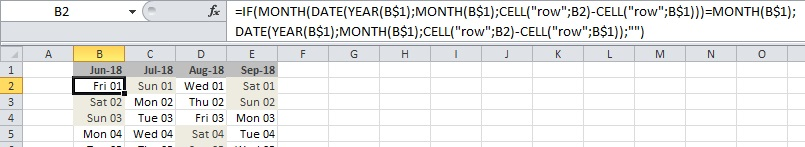Excel tricks

Written by pmd - - no comments

1- Generate a calendar in Excel

Here is how to generate a calendar in Excel. Using Excel 2010 in my case.

In cell B1 (by example), put a date :

01/06/2018

In cell B2 :

=IF(
MONTH(DATE(YEAR(B\$1);MONTH(B\$1);CELL("row";B2)-CELL("row";B\$1)))=MONTH(B\$1);
DATE(YEAR(B\$1);MONTH(B\$1);CELL("row";B2)-CELL("row";B\$1));"")

Then you just have to drag-n-drop down and right (or left).

To highlight saturdays and sundays :

=IF(ISBLANK(B2)=FALSE;OR(IF(WEEKDAY(B2)=7;TRUE;FALSE);IF(WEEKDAY(B2)=1;TRUE;FALSE));FALSE)

Here is the result :2- Number of days in one month

Put a date in cell B1. Then in another cell, formula is :

=DAY(DATE(YEAR(B1);MONTH(B1)+1;0))

It will return the number of days of the month in cell B1.